### CONTRAST Statement

CONTRAST ’label’ effect values <…effect values> </ options> ;

The CONTRAST statement enables you to perform custom hypothesis tests by specifying anvector or matrix for testing the univariate hypothesis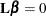or the multivariate hypothesis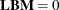. Thus, to use this feature you must be familiar with the details of the model parameterization that PROC GLM uses. For more information, see the section Parameterization of PROC GLM Models. All of the elements of thevector might be given, or if only certain portions of thevector are given, the remaining elements are constructed by PROC GLM from the context (in a manner similar to rule 4 discussed in the section Construction of Least Squares Means).

There is no limit to the number of CONTRAST statements you can specify, but they must appear after the MODEL statement. In addition, if you use a CONTRAST statement and a MANOVA, REPEATED, or TEST statement, appropriate tests for contrasts are carried out as part of the MANOVA, REPEATED, or TEST analysis. If you use a CONTRAST statement and a RANDOM statement, the expected mean square of the contrast is displayed. As a result of these additional analyses, the CONTRAST statement must appear before the MANOVA, REPEATED, RANDOM, or TEST statement.

In the CONTRAST statement,

label

identifies the contrast on the output. A label is required for every contrast specified. Labels must be enclosed in quotes.

effect

identifies an effect that appears in the MODEL statement, or the INTERCEPT effect. The INTERCEPT effect can be used when an intercept is fitted in the model. You do not need to include all effects that are in the MODEL statement.

values

are constants that are elements of thevector associated with the effect.

You can specify the following options in the CONTRAST statement after a slash (/).

E

displays the entirevector. This option is useful in confirming the ordering of parameters for specifying.

E=effect

specifies an error term, which must be one of the effects in the model. The procedure uses this effect as the denominator in F tests in univariate analysis. In addition, if you use a MANOVA or REPEATED statement, the procedure uses the effect specified by the E= option as the basis of the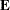matrix. By default, the procedure uses the overall residual or error mean square (MSE) as an error term.

ETYPE=n

specifies the type (1, 2, 3, or 4, corresponding to a Type I, II, III, or IV test, respectively) of the E= effect. If the E= option is specified and the ETYPE= option is not, the procedure uses the highest type computed in the analysis.

SINGULAR=number

tunes the estimability checking. If ABS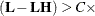number for any row in the contrast, thenis declared nonestimable.is the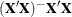matrix, and C is ABS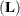except for rows whereis zero, and then it is 1. The default value for the SINGULAR= option is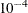. Values for the SINGULAR= option must be between 0 and 1.

As stated previously, the CONTRAST statement enables you to perform custom hypothesis tests. If the hypothesis is testable in the univariate case, SS(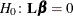) is computed as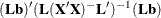where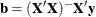. This is the sum of squares displayed on the analysis-of-variance table.

For multivariate testable hypotheses, the usual multivariate tests are performed using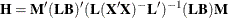where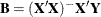and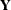is the matrix of multivariate responses or dependent variables. The degrees of freedom associated with the hypothesis are equal to the row rank of. The sum of squares computed in this situation is equivalent to the sum of squares computed using anmatrix with any row deleted that is a linear combination of previous rows.

Multiple-degrees-of-freedom hypotheses can be specified by separating the rows of thematrix with commas.

For example, for the model

proc glm;
class A B;
model Y=A B;
run;


with A at 5 levels and B at 2 levels, the parameter vector is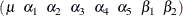To test the hypothesis that the pooled A linear and A quadratic effect is zero, you can use the followingmatrix: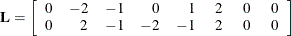The corresponding CONTRAST statement is

contrast 'A LINEAR & QUADRATIC'
a -2 -1  0  1  2,
a  2 -1 -2 -1  2;


If the first level of A is a control level and you want a test of control versus others, you can use this statement:

contrast 'CONTROL VS OTHERS'  a -1 0.25 0.25 0.25 0.25;


See the following discussion of the ESTIMATE statement and the section Specification of ESTIMATE Expressions for rules on specification, construction, distribution, and estimability in the CONTRAST statement.